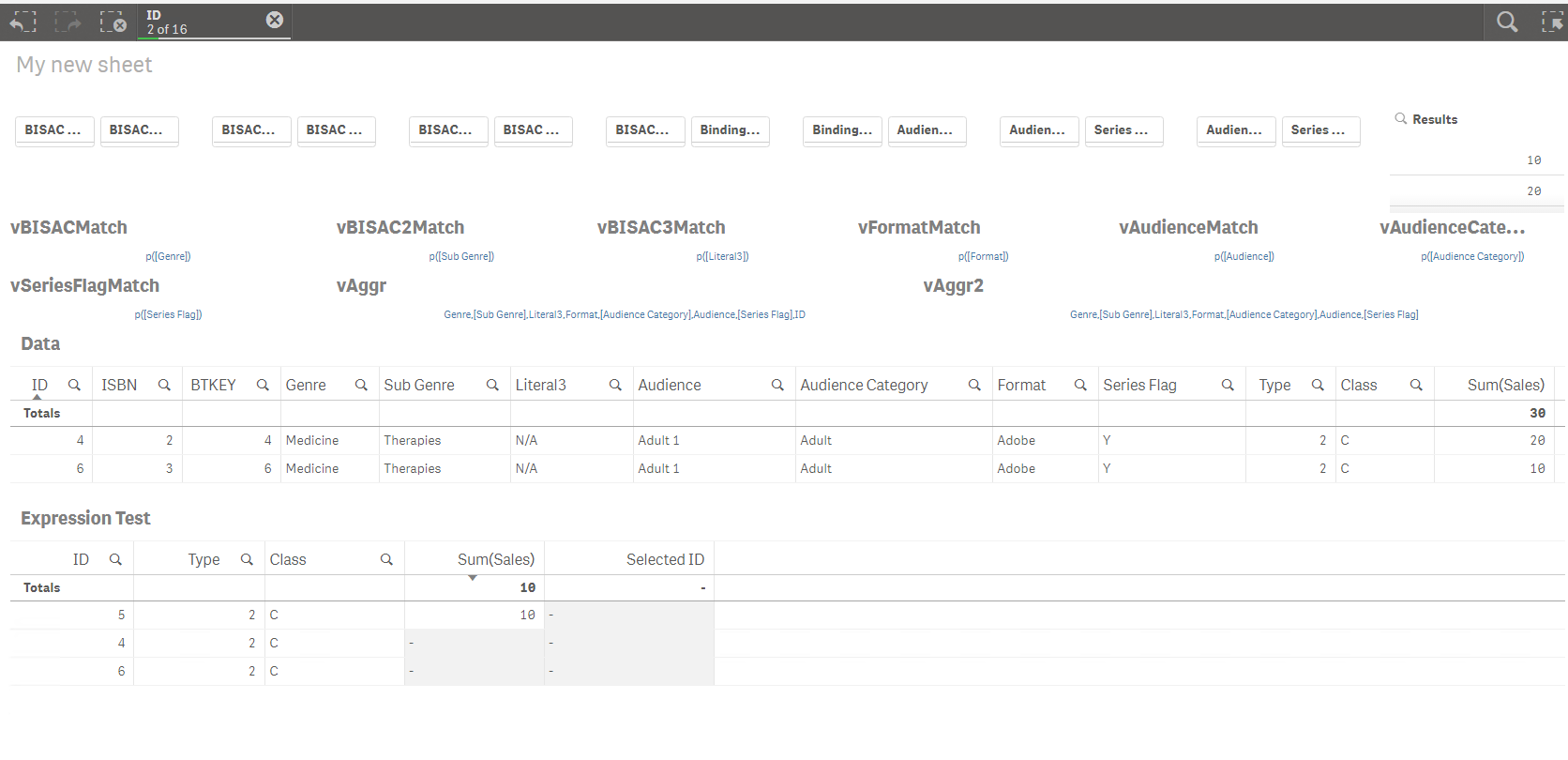# Qlik Sense App Development

Discussion board where members can learn more about Qlik Sense App Development and Usage.

Announcements
Qlik® Product Spotlight: Discover what’s possible. Get more from our products.
See for yourself. Register today.
Contributor

## Complex set analysis question please....

Hi there,

I select several ID's and return 2 ID's for each ID selected, where Type and Class value match the selected ID.

The below example shows the selection of ID's 1 and 4 to return:

ID's 2 and 3 (first two records with same possible values for Type and Class as ID 1

ID's 5 and 6 (first two records same possible values for Type and Class as ID 4

 Data ID Type Class Sales 1 1 A 10 2 1 A 10 3 1 A 10 4 2 C 20 5 2 C 30 6 2 C 10 7 2 C 21 8 3 D 34 9 3 D 21 10 4 D 10 Resulting Table ID Type Class Sales 2 1 A 10 3 1 A 10 5 2 C 30 6 2 C 10

I do this with,

If(Only({1<ID = p({<Type = p(Type), Class = p(Class), ID = e(ID)>})>}

Aggr(Rank(Only({1<ID = p({<Type = p(Type), Class = p(Class), ID = e(ID)>})>}-ID)), Type, Class, ID)) < 3,

Sum({1<ID = p({<Type = p(Type), Class = p(Class), ID = e(ID)>})>}[Sales]))

I would like to display the selected ID relevant to the result, such as...

 ID Type Class Sales Selected ID 2 1 A 10 1 3 1 A 10 1 5 2 C 30 4 6 2 C 10 4

Please can anyone offer some advice on the correct set analysis expression to use?

I have also attached a small test Qlik Sense QVF.

Thanks!!

Tags (2)
10 RepliesMVP

## Re: Complex set analysis question please....

Check my response here:

Complex set analysis question please....

If(Only({1<ID = p({<Type = p(Type), Class = p(Class), ID = e(ID)>})>}Aggr(Rank(Only({1<ID = p({<Type = p(Type), Class = p(Class), ID = e(ID)>})>}-ID)), Type, Class, ID)) < 3,

Only(TOTAL <Type, Class> ID))

Contributor

## Re: Complex set analysis question please....

awesome!!!!!Contributor

## Re: Complex set analysis question please....

Seems to work for one ID selection, but not for more than 1.

ThanksMVP

## Re: Complex set analysis question please....

Did you look at the screenshot hereContributor

## Re: Complex set analysis question please....

Apologies, yes I can see that now, and works perfectly.

When I migrate into my application, I get no results for more than one selection, but I am using dynamic sets and aggregations.

I understand this surpasses the remit of this question which you have correctly answered, but have attached the application should you wish to take a look.

The user selects from the '* Match' filters to dynamically change the set analysis between p(field) and {'*'}

This also then dynamically changes the aggregation, where if {'*'}, this is removed from the aggregation field string.

Thanks again

Contributor

## Re: Complex set analysis question please....

QVF with dynamic sets / aggregation.

ThanksMVP

## Re: Complex set analysis question please....

Try this

=If(Only({1<ID = p({<Genre =  (\$(=\$(vBISACMatch))), [Sub Genre] = (\$(=\$(vBISAC2Match))),

Literal3 = (\$(=\$(vBISAC3Match))),Format = (\$(=\$(vFormatMatch))),Audience = (\$(=\$(vAudienceMatch))),

[Audience Category] = (\$(=\$(vAudienceCategoryMatch))),[Series Flag] = (\$(=\$(vSeriesFlagMatch))),

ID = e(ID)>})>}Aggr(Rank(Only({1<ID = p({<Genre =  (\$(=\$(vBISACMatch))), [Sub Genre] = (\$(=\$(vBISAC2Match))),

Literal3 = (\$(=\$(vBISAC3Match))),Format = (\$(=\$(vFormatMatch))),Audience = (\$(=\$(vAudienceMatch))),

[Audience Category] = (\$(=\$(vAudienceCategoryMatch))),[Series Flag] = (\$(=\$(vSeriesFlagMatch))),

ID = e(ID)>})>}-ID)), \$(vAggr))) <= \$(vResults),

Only(TOTAL <Type, Class> ID))

Contributor

## Re: Complex set analysis question please....

Thanks,

This seems to work ok, until you select two ID's with the same

Genre,

Sub Genre,

Literal3,

Audience,

Audience Category,

Format,

Series Flag,

Type,

Class

I have tried incorporating BTKEY / ISBN into the expression, but this doesn't seem to resolve the above issue...

=If(Only({1<ID = p({<Genre =  (\$(=\$(vBISACMatch))), [Sub Genre] = (\$(=\$(vBISAC2Match))),

Literal3 = (\$(=\$(vBISAC3Match))),Format = (\$(=\$(vFormatMatch))),Audience = (\$(=\$(vAudienceMatch))),

[Audience Category] = (\$(=\$(vAudienceCategoryMatch))),[Series Flag] = (\$(=\$(vSeriesFlagMatch))),

ID = e(ID)>})>}Aggr(Rank(Only({1<ID = p({<Genre =  (\$(=\$(vBISACMatch))), [Sub Genre] = (\$(=\$(vBISAC2Match))),

Literal3 = (\$(=\$(vBISAC3Match))),Format = (\$(=\$(vFormatMatch))),Audience = (\$(=\$(vAudienceMatch))),

[Audience Category] = (\$(=\$(vAudienceCategoryMatch))),[Series Flag] = (\$(=\$(vSeriesFlagMatch))),

ID = e(ID)>})>}-ID)), \$(vAggr))) <= \$(vResults),

Only(TOTAL <Type, Class, BTKEY, ISBN> ID))

Thanks AgainMVP

## Re: Complex set analysis question please....

You want same id based on Type and Class... where did these other fields came in from? I am not sure what your final goal is? Do you mind the overall goal?

Community Browser